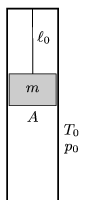Mathematical and Physical Journal
for High Schools
Issued by the MATFUND Foundation
 Already signed up? New to KöMaL?

#Problem P. 4302. (December 2010)

P. 4302. There is some Oxygen gas in a vertical, long enough, metal, cylinder, above a piston of mass m=60 kg, and of cross section A=2 dm2. The cylinder is closed at its top and the piston hangs on a thread of length0=11.2 dm. The initial pressure and temperature of the gas are equal to the pressure and temperature outside the cylinder, p0=105 Pa T0=273 K, respectively. The wall of the cylinder is a good heat conductor, and the piston can move in the cylinder without friction. By what amount will the total energy of the system change if the thread breaks?(4 pont)

Deadline expired on January 10, 2011.

Sorry, the solution is available only in Hungarian. Google translation

Megoldás. Mivel a gáz hőmérséklete a kezdeti és végállapotban megegyezik, csak a dugattyú helyzeti energiája változik, mégpedig $\displaystyle \Delta E=-\frac{mgl_0}{\frac{p_0 A}{mg}-1}$ értékkel.

### Statistics:

 115 students sent a solution. 4 points: 83 students. 3 points: 5 students. 2 points: 22 students. 1 point: 2 students. 0 point: 1 student. Unfair, not evaluated: 2 solutionss.

Problems in Physics of KöMaL, December 2010